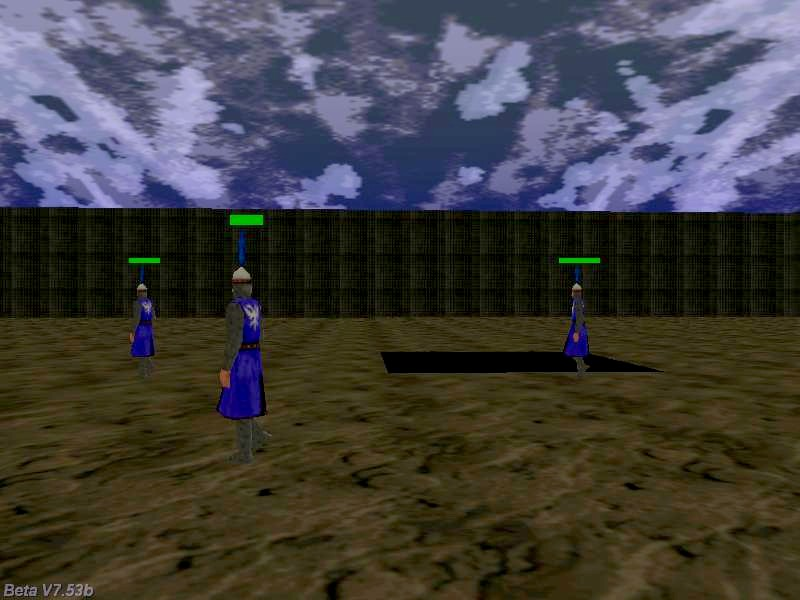Questions from the forum
 Q: Is it possible to play a movie inside the level when the player comes close to a certain entity (the exit gate)? A: Sure, take a look at the code below.   // attach this action to a sprite that has the same size with your movie action movie_gate() {        // wait until the player entity is loaded in the level        while (!player) {wait (1);}        // wait until the player comes close to the exit gate        while (vec_dist(player.x, my.x) > 150) {wait (1);}        // now play the movie file        media_play("mymovie.avi", bmap_for_entity (my, 0), 100); // use your own movie name here }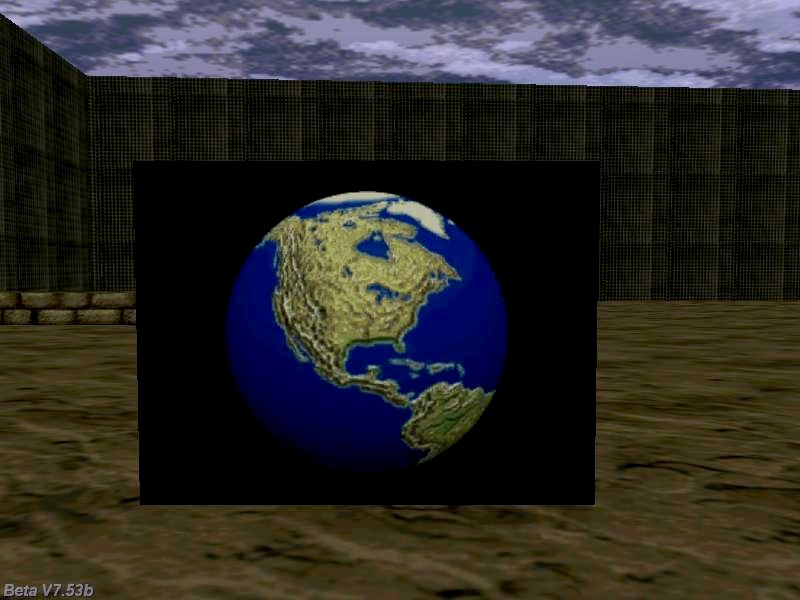Q: How can I generate random passwords using lite-C? A: Here's an example.   var password_length = 8; // generate passwords that contain 8 characters, play with this number   STRING* password_str = "#30"; // stores passwords with up to 30 characters STRING* temp_str = " ";   FONT* arial_font = "Arial#20";   TEXT* password_txt = {        pos_x = 20;        pos_y = 20;        font = arial_font;        string(password_str);        flags = VISIBLE; }   // press any key to generate a new password function passwords_startup() {        var i, temp;        randomize();        while (1)        {                while (key_any) {wait (1);} // wait until all the keys are released                while (!key_any) {wait (1);} // wait until a key is pressed                str_cpy(password_str, "#30"); // reset password_str                i = 0;                while (i < password_length) // generate a number of 8 characters for our password                {                        // generate a random ascii code (visible, printable characters only)                        temp = integer(random(95)) + 32;                        str_for_asc(temp_str, temp); // convert the random number to its string equivalent                        str_cat(password_str, temp_str);                        i++;                }        } }     Q: I'd like to create a 2D arkanoid clone, but I hate the idea of defining hundreds of panels. Isn't there an easier way? A: Use pan_create to create and place your panels easily.   PANEL* temp_pan;   // these numerical values work fine for a screen resolution of 800 x 600 pixels function aliens_startup() {        var temp1 = 30; // temp1 gives the coordinates of the first brick on the x axis        var temp2 = 20; // temp2 gives the coordinates of the first brick on the y axis        // create rows of bricks until their y exceeds 300        while (temp2 < 300)        {                // generate bricks until their x exceeds 760                while (temp1 < 760)                {                        // block.pcx has 32 x 16 pixels in my example, but you can use any other size for the bitmap                        temp_pan = pan_create("bmap = block.pcx; flags = overlay, visible;", 10);                        temp_pan.pos_x = temp1;                        temp_pan.pos_y = temp2;                        temp1 += 50;                }                temp1 = 30;                temp2 += 40;        } }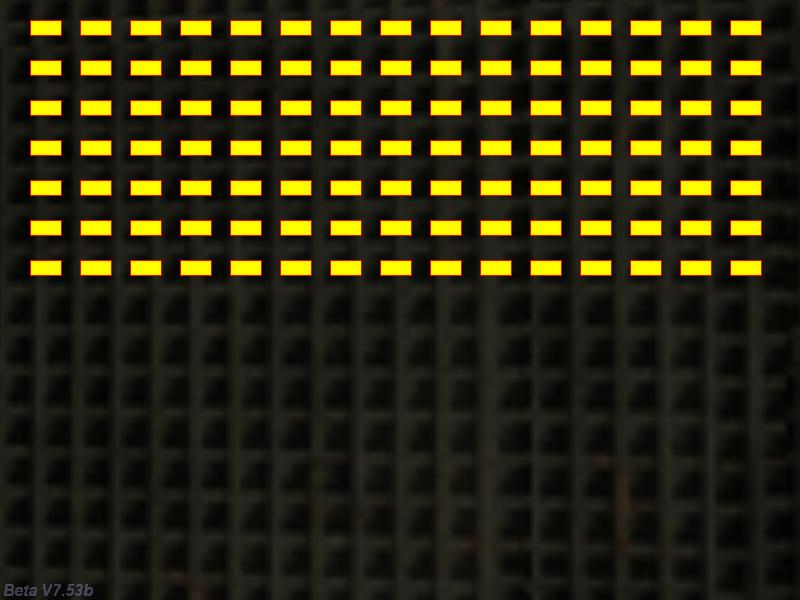Q: My player model has 3 animations: "walk", "stand" and "look". I'd like it to "walk" when the movement keys are played, "stand" when it stops and "look" after a longer period of inactivity. How can I do that? A: Use this player / camera example as a base for your code.   action players_code() {        VECTOR temp;        VECTOR movement_speed; // player's movement speed        var anim_percentage; // animation percentage        var distance_to_ground;        var time_passed = 0;        player = my; // I'm the player        while (1)        {                my.pan += 6 * (key_a - key_d) * time_step; // rotate the player using the "A" and "D" keys                vec_set (temp.x, my.x); // copy player's position to temp                temp.z -= 10000; // set temp.z 10000 quants below player's origin                distance_to_ground = c_trace (my.x, temp.x, IGNORE_ME | USE_BOX);                movement_speed.x = 5 * (key_w - key_s) * time_step; // move the player using "W" and "S"                movement_speed.y = 0; // don't move sideways                movement_speed.z = -(distance_to_ground - 20); // 20 = experimental value                c_move (my, movement_speed.x, nullvector, GLIDE); // move the player                if (!key_w && !key_s) // the player isn't moving?                {                        time_passed += time_step / 16; // add "1" to time_passed every second                        if (time_passed >= 20) // at least 20 seconds have passed?                        {                                anim_percentage += 3 * time_step; // 3 = "look" animation speed                                ent_animate(my, "look", anim_percentage, ANM_CYCLE); // then play the "look" animation                        }                        else // the player is standing                        {                                anim_percentage += 2 * time_step; // 2 = "stand" animation speed                                ent_animate(my, "stand", anim_percentage, ANM_CYCLE);                        }                }                else // the player is moving?             {                        time_passed = 0;                     ent_animate(my, "walk", anim_percentage, ANM_CYCLE); // play the "walk" animation                        anim_percentage += 5 * time_step; // 5 = "walk" animation speed                }                // camera code                camera.x = player.x - 250 * cos(player.pan);                camera.y = player.y - 250 * sin(player.pan); // use the same value (250) here                camera.z = player.z + 150; // place the camera above the player, play with this value                camera.tilt = -20; // look down at the player                camera.pan = player.pan;                wait (1);        } }     Q: I'd like my npc to move from A to B, stopping at certain points on the path for a specified number of seconds. How can I do that? A:  Place your npc in the level and assign it the action named "patroller". Create a virtual path from A to B using small entities, attach them the action named "target_init" and set their skill1 to the waiting time (in seconds), if needed.   ENTITY* my_target;   action patroller() {        VECTOR temp;        while (1)        {                // scan for camera positions that are placed up to 1000 quants away from this entity                c_scan(my.x, my.pan, vector(360, 60, 1000), IGNORE_ME | SCAN_ENTS | SCAN_LIMIT);                if (you) // a target was detected?                {                        my_target = you; // the closest position to the npc is set to "you" by c_scan                        my_target.scale_z = 4;                        vec_set(temp, my_target.x);                        vec_sub(temp, my.x);                        vec_to_angle(my.pan, temp); // rotate the patroller towards the position                        my.tilt = 0; // but don't allow it to change its tilt angle                        // the patroller looks at its target now                        while (vec_dist (my.x, my_target.x) > 50) // the patroller moves until it comes close to the target                        {                                my.skill22 += 5 * time_step; // 5 gives the "walk" animation speed                                c_move(my, vector(5 * time_step, 0, 0), nullvector, IGNORE_PASSABLE | GLIDE); // 5 = movement speed                                ent_animate(my, "walk", my.skill22, ANM_CYCLE);                                wait (1);                        }                        // the patroller has come close to target here                        my.skill99 = 0;                        while (my.skill99 < my_target.skill1)                        {                                my.skill99 += time_step / 16;                                ent_animate(my, "stand", my.skill22, ANM_CYCLE);                                my.skill22 += 3 * time_step; // 3 gives the "stand" animation speed                                wait (1);                        }                        ent_remove(my_target); // remove the current target and prepare for the following target                }                else // reached the end of the path?                {                        ent_animate(my, "stand", my.skill22, ANM_CYCLE); // then default to standing                        my.skill22 += 3 * time_step; // 3 gives the "stand" animation speed                }                wait (1);        } }   action target_init() {        set (my, PASSABLE | INVISIBLE); // player's movement code should ignore passable entities        my.emask |= ENABLE_SCAN; // the target entity is sensitive to c_scan instructions }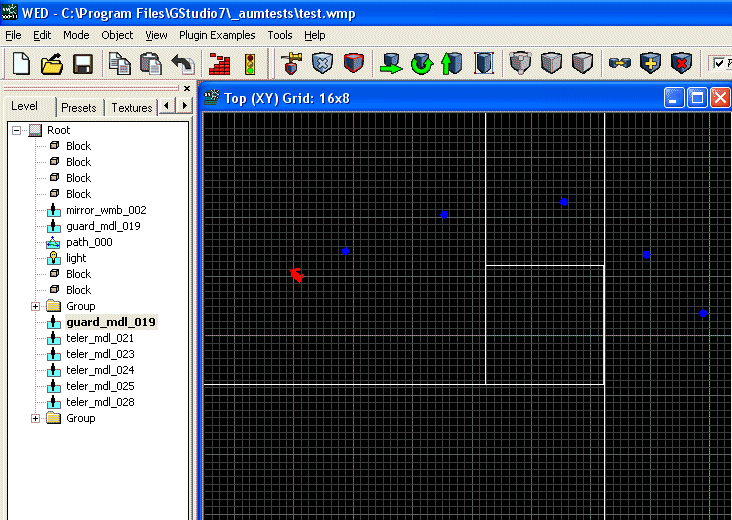Q: I'd like to have some targets (sprites) that rotate around their pan angles when they are shot. Can you help? A: Sure; use the code below.   SOUND* bullet_wav = "bullet.wav";   function fire_bullets() {        VECTOR trace_coords;        vec_set(trace_coords.x, vector(5000, 0, 0)); // trace 5000 quants in front of the player        // rotate "trace_coords"        vec_rotate(trace_coords.x, camera.pan);        vec_add(trace_coords.x, player.x); // add the resulting vector to player's position        snd_play(bullet_wav, 70, 0); // play the bullet sound        if (c_trace (player.x, trace_coords.x, IGNORE_ME + USE_BOX) > 0) // the "c_trace" ray has hit something?        {                if (you) // and the hit object is an entity?                {                        if (you.skill1 == 99) // and the hit entity has its skill1 set to 99?                        {                                you.skill50 = 30; // 30 = maximum rotation speed                        }                }        } }   action players_code() {        on_mouse_left = fire_bullets;        player = my; // I'm the player        set (my, INVISIBLE); // no need to see player's model in 1st person mode        while (1)        {                // move the player using the "W", "S", "A" and "D" keys; "10" = movement speed, "6" = strafing speed                c_move (my, vector(10 * (key_w - key_s) * time_step, 6 * (key_a - key_d) * time_step, 0), nullvector, GLIDE);                vec_set (camera.x, player.x); // use player's x and y for the camera as well                camera.z += 30; // place the camera 30 quants above the player on the z axis (approximate eye level)                camera.pan -= 5 * mouse_force.x * time_step; // rotate the camera around by moving the mouse                camera.tilt += 3 * mouse_force.y * time_step; // on its x and y axis                player.pan = camera.pan; // the camera and the player have the same pan angle                wait (1);        } }   action shooting_target() {        my.skill1 = 99; // this entity will rotate around its axis        while (1)        {                while (my.skill50 <= 0) {wait (1);} // the entity waits until it is shot by the player                while (my.skill50 > 0)                {                        my.pan += my.skill50 * time_step; // rotate the entity around its pan angle                        my.skill50 -= 0.5 * time_step; // decrease the rotation speed as the time passes                        wait (1);                }        } }Q: How can I see the c_scan range for an entity? I'd like to have some visual pointers, if possible. A: Get the triangle.mdl from this months' "Resources" and use it together with the code below; it displays the horizontal scanning sector and the scanning distance.   function scan_view() {        proc_kill(4); // follow the entity smoothly        set(my, PASSABLE | TRANSLUCENT);        my.alpha = 20;        while (you)        {                my.x = you.x;                my.y = you.y;                my.z = you.z - 35; // move the triangle at the floor level                my.pan = you.pan;                my.tilt = you.tilt;                my.scale_x = you.skill3 / 10; // set the proper scale_x and scale_y for the triangle model                my.scale_y = 2 * you.skill3 * tan(you.skill1 / 2) / 10; // scale_y = 2 * d * tg (alpha / 2)                wait (1);        } }   action my_entity() // simple entity code, makes it rotate around while scanning the area {        my.skill1 = 30; // horizontal angle        my.skill2 = 20; // vertical angle        my.skill3 = 200; // scanning range        VECTOR temp;        // create the triangular area using a triangle that has a size of 10 pixels        ent_create("triangle.mdl", nullvector, scan_view);        while (1)        {                c_scan(my.x, my.pan, my.skill1, IGNORE_ME | SCAN_ENTS | SCAN_LIMIT);                c_move(my, vector(5 * time_step, 0, 0), nullvector, IGNORE_PASSABLE | GLIDE); // 5 = movement speed                my.skill22 += 5 * time_step; // 5 gives the "walk" animation speed                my.pan += 1 * time_step;                ent_animate(my, "walk", my.skill22, ANM_CYCLE);                wait (1);        } }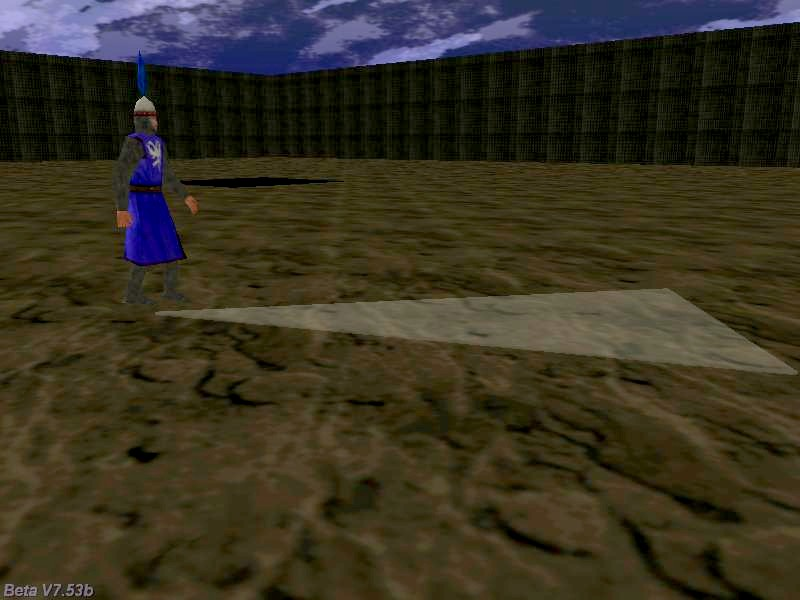Q: I've been playing Guild Wars and I have noticed that they have intelligent NPCs that walk, stop in front of walls, and then change their directions and move away. Could something similar be achieved using GameStudio? A: Take a look at the code below; it does exactly what you need.   action intelligent_npc() // stops in front of the wall, and then changes its direction {        VECTOR front_pos;        var distance_covered;        while (1)        {                vec_set(front_pos.x, vector(50, 0, 0)); // compute a vector that's 50 quants in front of the player                // rotate "front_pos" in the direction (angles) given by the entity                vec_rotate(front_pos.x, my.pan);                vec_add(front_pos.x, my.x); // add the resulting vector to entity's position                if (c_content(front_pos.x, 0) == 1) // front_pos isn't touching any walls?                {                        // 5 gives the movement speed                        distance_covered = c_move(my, vector(5 * time_step, 0, 0), nullvector, IGNORE_PASSABLE | GLIDE);                        my.skill22 += 5 * time_step; // 5 gives the "walk" animation speed                        ent_animate(my, "walk", my.skill22, ANM_CYCLE);                }                else // the player is close to a wall now?                {                        my.skill99 = 0;                        while (my.skill99 < 5) // stays in front of the wall for 5 seconds                        {                                my.skill99 += time_step / 16;                                ent_animate(my, "stand", my.skill22, ANM_CYCLE);                                my.skill22 += 3 * time_step; // 3 gives the "stand" animation speed                                wait (1);                        }                        my.skill99 = my.pan;                        my.skill99 += random(180);                        while (my.pan < my.skill99)                        {                                my.pan += 5 * time_step;                                wait (1);                        }                }                wait (1);        } }Q: I've got the player surrounded by enemies, but I'm having a hard time trying to detect what enemy the player is facing. Can you help me please? A: The following snippet computes the pan angle difference between each enemy and the player. When this difference is in the 150...210 degrees range (180 + or - 30 degrees) that enemy is facing the player; the code sets scale_z to 1.5 for that particular enemy.   action player_code() // attach this action to your player {        var movement_speed = 10; // movement speed        VECTOR temp;        player = my; // I'm the player        while (1)        {                temp.x = movement_speed * (key_cuu - key_cud) * time_step;                temp.y = 0;                temp.z = 0;                my.pan += 4 * (key_cul - key_cur) * time_step;                c_move (my, temp.x, nullvector, IGNORE_PASSABLE | GLIDE);                wait (1);        } }   // simple enemy action; surround the player with several models that have this action attached to them action my_enemy() {        VECTOR temp;        while (!player) {wait (1);}        while (1)        {                vec_set(temp, player.x);                vec_sub(temp, my.x);                vec_to_angle(my.pan, temp); // rotate the enemy towards the player                my.tilt = 0; // but don't allow it to change its tilt angle                if (ang(my.pan - player.pan) > 150)                {                        // the angle between the enemy and the player is in the 150...210 degrees range?                        if (ang(my.pan - player.pan) < 210)                        {                                // then increase the z scale of the entity that is facing the player                                my.scale_z = 1.5;                        }                }                else // the player isn't facing this enemy                {                        my.scale_z = 1; // so restore the initial scale_z value                }                wait (1);        } }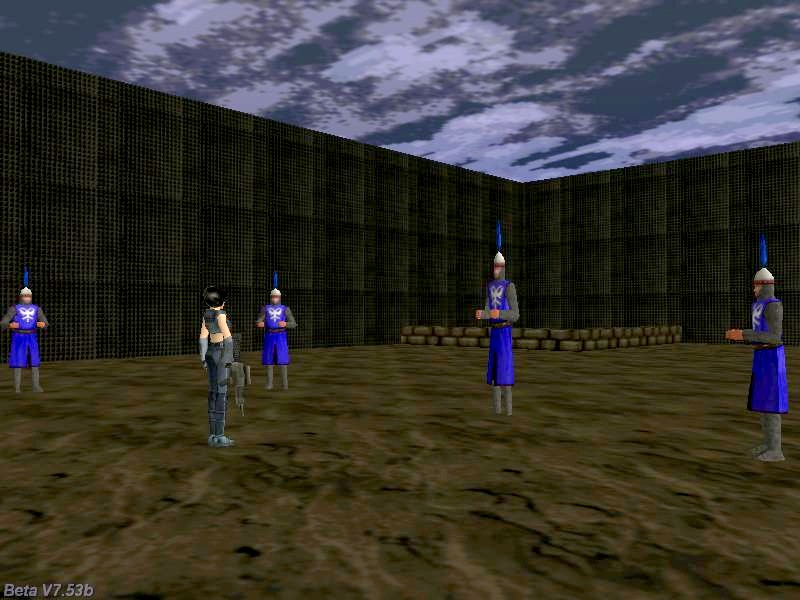Q: Please give an example of showing local variables as panels over entities; there is too much confusion with this in the forum. Example: you have one action attached to all enemies and you want to see the health panel over them? A: The code below uses a green sprite of 4 x 4 pixels to create a health panel above each entity. The health value is read from a local variable (entity's skill20).   #define health skill20   function health_indicator() {        set (my, PASSABLE);        while (you)        {                vec_set (my.x, you.x);                my.z += 60;                my.scale_x = you.health * 0.1; // skill20 aka "health" stores the health for each entity                wait (1);        } }   action my_enemy() // simple enemy action, makes it rotate in a circle {        my.health = 10 + random(90);        ent_create ("health.pcx", nullvector, health_indicator);        while (1)        {                c_move(my, vector(5 * time_step, 0, 0), nullvector, IGNORE_PASSABLE | GLIDE); // 5 = movement speed                my.skill22 += 5 * time_step; // 5 gives the "walk" animation speed                my.pan += 1 * time_step;                ent_animate(my, "walk", my.skill22, ANM_CYCLE);                wait (1);        } }   function randomize_startup() {        randomize(); }